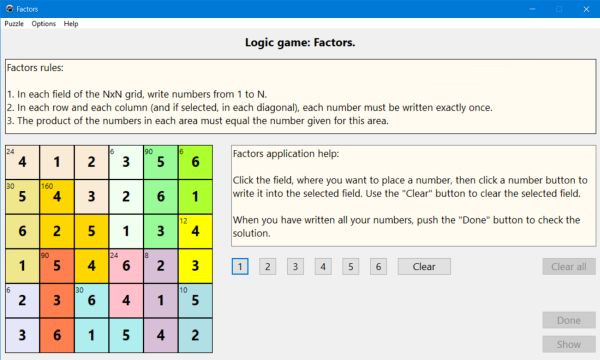# Computing: Free Pascal Programming

 Programming Home Home Contact

## Logic number puzzle: Factors (Factors).

Description: Puzzle game with numbers to be placed onto a grid. The rules of Factors are as follows:
1. In each field of the NxN grid, write numbers from 1 to N.
2. In each row and each column (and if selected, in each diagonal), each number must be written exactly once.
3. The product of the numbers in each area must equal the number given for this area.
The game is a typical logic game: In order to find the correct solution of the puzzle, you have to explore the product values, and by logical thinking (concluding from what you know, that a given field must be a given number, or on the contrary, can't be a given number), determine, to which fields which numbers have to be written. "Factors" is essentially the same game as Sumdoku, except that with "Sumdoku", the sums (and not the products) of the areas are displayed.
The game is played exclusively with the mouse: Click the field, where you want to write a number, then click one of the number buttons to write the corresponding number or "Clear" to remove the number from the actually selected field.

Note: The "unique numbers on diagonals" rule is not implemented in "Factors" version 1.0.

Free Pascal features: Changing the color of shapes and the caption of static-texts and labels during execution. Creating objects during runtime. Two-dimensional arrays and array indexed by array elements (classic Pascal).

Screenshot: